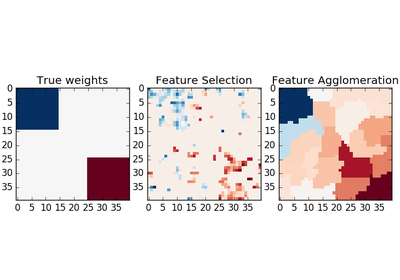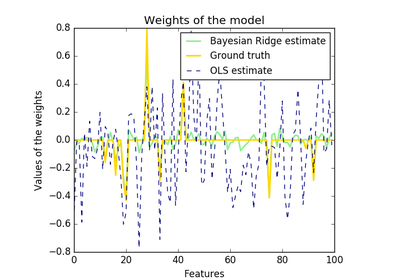# `sklearn.linear_model`.BayesianRidge¶

class `sklearn.linear_model.``BayesianRidge`(n_iter=300, tol=0.001, alpha_1=1e-06, alpha_2=1e-06, lambda_1=1e-06, lambda_2=1e-06, compute_score=False, fit_intercept=True, normalize=False, copy_X=True, verbose=False)[source]

Bayesian ridge regression

Fit a Bayesian ridge model and optimize the regularization parameters lambda (precision of the weights) and alpha (precision of the noise).

Read more in the User Guide.

Parameters: n_iter : int, optional Maximum number of iterations. Default is 300. tol : float, optional Stop the algorithm if w has converged. Default is 1.e-3. alpha_1 : float, optional Hyper-parameter : shape parameter for the Gamma distribution prior over the alpha parameter. Default is 1.e-6 alpha_2 : float, optional Hyper-parameter : inverse scale parameter (rate parameter) for the Gamma distribution prior over the alpha parameter. Default is 1.e-6. lambda_1 : float, optional Hyper-parameter : shape parameter for the Gamma distribution prior over the lambda parameter. Default is 1.e-6. lambda_2 : float, optional Hyper-parameter : inverse scale parameter (rate parameter) for the Gamma distribution prior over the lambda parameter. Default is 1.e-6 compute_score : boolean, optional If True, compute the objective function at each step of the model. Default is False fit_intercept : boolean, optional whether to calculate the intercept for this model. If set to false, no intercept will be used in calculations (e.g. data is expected to be already centered). Default is True. normalize : boolean, optional, default False If True, the regressors X will be normalized before regression. This parameter is ignored when fit_intercept is set to False. When the regressors are normalized, note that this makes the hyperparameters learnt more robust and almost independent of the number of samples. The same property is not valid for standardized data. However, if you wish to standardize, please use preprocessing.StandardScaler before calling fit on an estimator with normalize=False. copy_X : boolean, optional, default True If True, X will be copied; else, it may be overwritten. verbose : boolean, optional, default False Verbose mode when fitting the model. coef_ : array, shape = (n_features) Coefficients of the regression model (mean of distribution) alpha_ : float estimated precision of the noise. lambda_ : float estimated precision of the weights. scores_ : float if computed, value of the objective function (to be maximized)

Notes

See examples/linear_model/plot_bayesian_ridge.py for an example.

Examples

```>>> from sklearn import linear_model
>>> clf = linear_model.BayesianRidge()
>>> clf.fit([[0,0], [1, 1], [2, 2]], [0, 1, 2])
...
BayesianRidge(alpha_1=1e-06, alpha_2=1e-06, compute_score=False,
copy_X=True, fit_intercept=True, lambda_1=1e-06, lambda_2=1e-06,
n_iter=300, normalize=False, tol=0.001, verbose=False)
>>> clf.predict([[1, 1]])
array([ 1.])
```

Methods

 `decision_function`(\*args, \*\*kwargs) DEPRECATED: and will be removed in 0.19. `fit`(X, y) Fit the model `get_params`([deep]) Get parameters for this estimator. `predict`(X) Predict using the linear model `score`(X, y[, sample_weight]) Returns the coefficient of determination R^2 of the prediction. `set_params`(\*\*params) Set the parameters of this estimator.
`__init__`(n_iter=300, tol=0.001, alpha_1=1e-06, alpha_2=1e-06, lambda_1=1e-06, lambda_2=1e-06, compute_score=False, fit_intercept=True, normalize=False, copy_X=True, verbose=False)[source]
`decision_function`(*args, **kwargs)[source]

DEPRECATED: and will be removed in 0.19.

Decision function of the linear model.

Parameters: X : {array-like, sparse matrix}, shape = (n_samples, n_features) Samples. C : array, shape = (n_samples,) Returns predicted values.
`fit`(X, y)[source]

Fit the model

Parameters: X : numpy array of shape [n_samples,n_features] Training data y : numpy array of shape [n_samples] Target values self : returns an instance of self.
`get_params`(deep=True)[source]

Get parameters for this estimator.

Parameters: deep : boolean, optional If True, will return the parameters for this estimator and contained subobjects that are estimators. params : mapping of string to any Parameter names mapped to their values.
`predict`(X)[source]

Predict using the linear model

Parameters: X : {array-like, sparse matrix}, shape = (n_samples, n_features) Samples. C : array, shape = (n_samples,) Returns predicted values.
`score`(X, y, sample_weight=None)[source]

Returns the coefficient of determination R^2 of the prediction.

The coefficient R^2 is defined as (1 - u/v), where u is the regression sum of squares ((y_true - y_pred) ** 2).sum() and v is the residual sum of squares ((y_true - y_true.mean()) ** 2).sum(). Best possible score is 1.0 and it can be negative (because the model can be arbitrarily worse). A constant model that always predicts the expected value of y, disregarding the input features, would get a R^2 score of 0.0.

Parameters: X : array-like, shape = (n_samples, n_features) Test samples. y : array-like, shape = (n_samples) or (n_samples, n_outputs) True values for X. sample_weight : array-like, shape = [n_samples], optional Sample weights. score : float R^2 of self.predict(X) wrt. y.
`set_params`(**params)[source]

Set the parameters of this estimator.

The method works on simple estimators as well as on nested objects (such as pipelines). The latter have parameters of the form `<component>__<parameter>` so that it’s possible to update each component of a nested object.

Returns: self :

## Examples using `sklearn.linear_model.BayesianRidge`¶Feature agglomeration vs. univariate selectionBayesian Ridge Regression